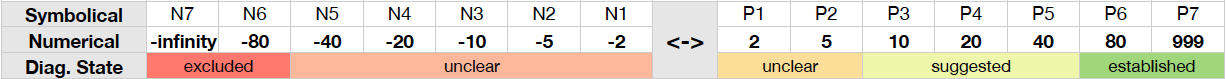## Definition#

Diagnosis rules are rules that assign scorepoints to diagnoses, which leads to a valuation of the diagnoses at the end of the problem-solving process. Basically, each diagnosis has a 'score account' that is initially set to 0. If diagnosis rules 'fire', that is if their condition part evaluates to true, they add a certain value to a diagnosis. If such a diagnosis attains a certain threshold value (in d3web this is 42) it rates as established.

## Syntax#

```IF (rc)
THEN diag1 = P3 ```
The example shows the basic notation of diagnosis rules. If condition rc (which can either be a simple or a combined condition) evaluated to true the value P3 is added to the score account of diag1.
Another example how a diagnosis rule can look like:
```%%Rule
IF question_oc = answer_oc AND question_num > 10
THEN diagnosis = P3
%```

Also the keywords suggested, excluded and established can be used:

```%%Rule
IF solution = established
THEN diagnosis = 1
%```

```%%Rule
IF solution = excluded
THEN diagnosis = established
%```

## Scorepoints#

To ease the formalization of diagnosis rules, certain symbolical score categories are used instead of numerical values. The mapping between numerical values, symbolical categories, and resulting diagnosis states is presented in the following table: